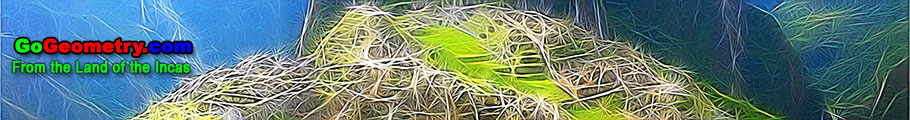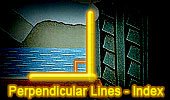Perpendicular Lines, Theorems and Problems - Table of Content  (19)Proposed Problem 112. Area of square and triangle. Perpendicular lines. The Pythagorean Curiosity Triangles and squares, fifteen conclusions. Perpendicular lines, Parallel lines. Triangle and Squares Theorem 1: with Interactive Geometry Software Step-by-Step construction, Manipulation, and animation. Perpendicular lines, Parallel lines. Proposed Problem 91. Similar Triangles, Altitude, Perpendicular lines, Parallel lines. Taylor Circle Theorem Using TracenPoche Dynamic Software Step-by-Step construction, Manipulation, and animation, Perpendicular lines. Henry Martyn Taylor and the blind student of mathematics Proposed Problem 78: Angles of a Circle. Perpendicular and parallel lines, Midpoint, Diameter, Chord, Cyclic quadrilateral, Congruence. Proposed Problem 76: Area of a Circle. Square, Circle, Circular Sector, Perpendicular lines. Interactive Orthopole of a Triangle: Using TracenPoche Dynamic Geometry Software, Online Step-by-Step construction, manipulation, and animation, Perpendicular lines. Proposed Problem 70: Squares inscribed in a triangle, Similarity. Perpendicular lines, Parallel lines. Proposed Problem 69: Square inscribed in a triangle, Similarity. Perpendicular lines, Parallel lines. Go to Page: Previous| 1 | 2 | 3 | 4 | 5 | 6 | 7 | 8 | 9 | 10 | 11 | 12 | 13 | 14 | 15 | 16 | 17 | 18 | 19 | 20 | Next

 Home | Search | Geometry | Post a comment | Email | Post a comment | By Antonio Gutierrez Last updated: Feb 15, 2015